Chapter 15, Problem 4SEB

Chapter
Section
Textbook Problem

FINANCIAL RATIOS Based on the financial statements, shown on pages 603–604, for McDonald Carpeting Co. (income statement, statement of owner’s equity, and balance sheet), prepare the following financial ratios. All sales are credit sales. The balance of Accounts Receivable on January 1, 20--, was $6,800. 1. Working capital 2. Current ratio 3. Quick ratio 4. Return on owner’s equity 5. Accounts receivable turnover and average number of days required to collect receivables 6. Inventory turnover and average number of days required to sell inventory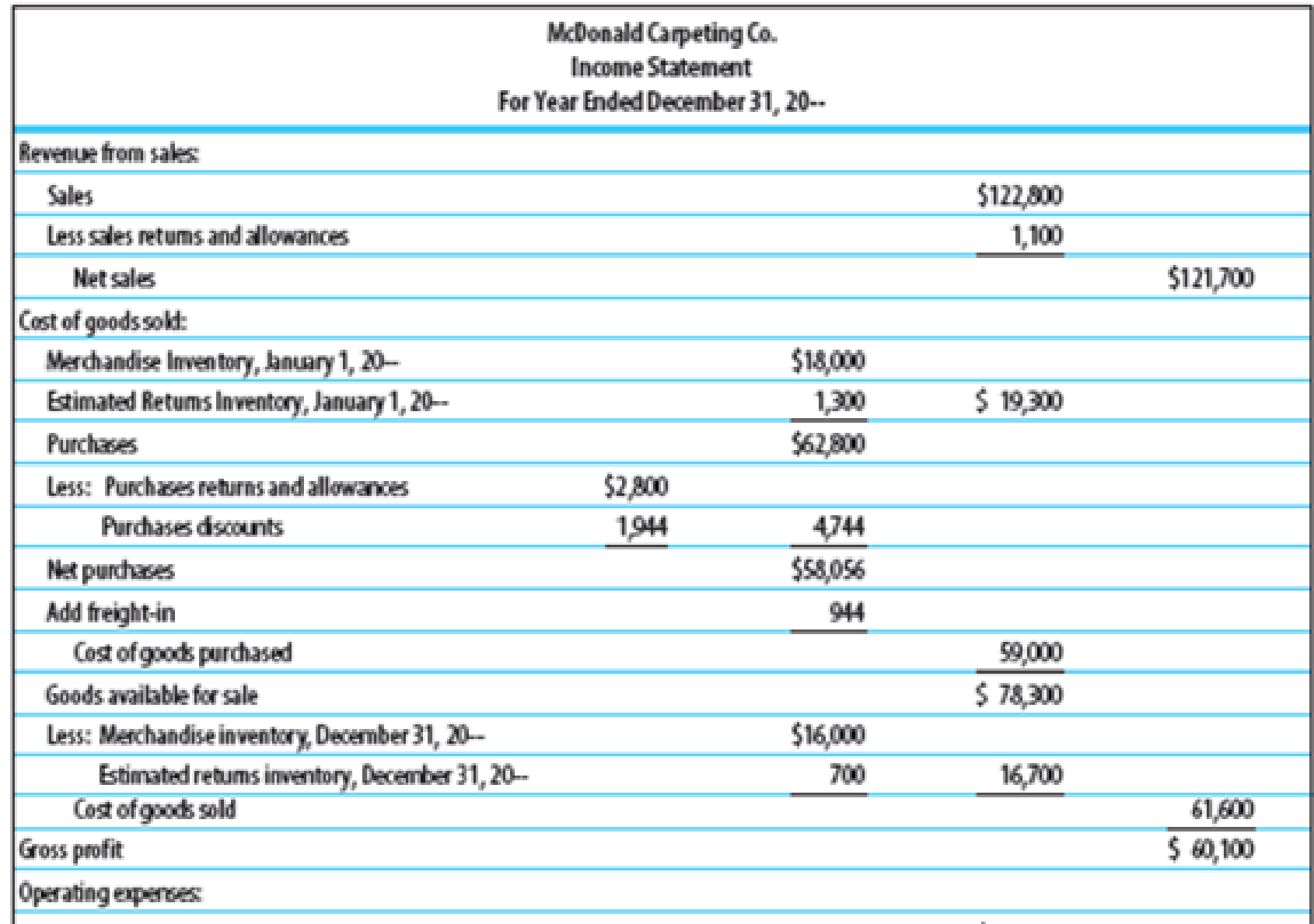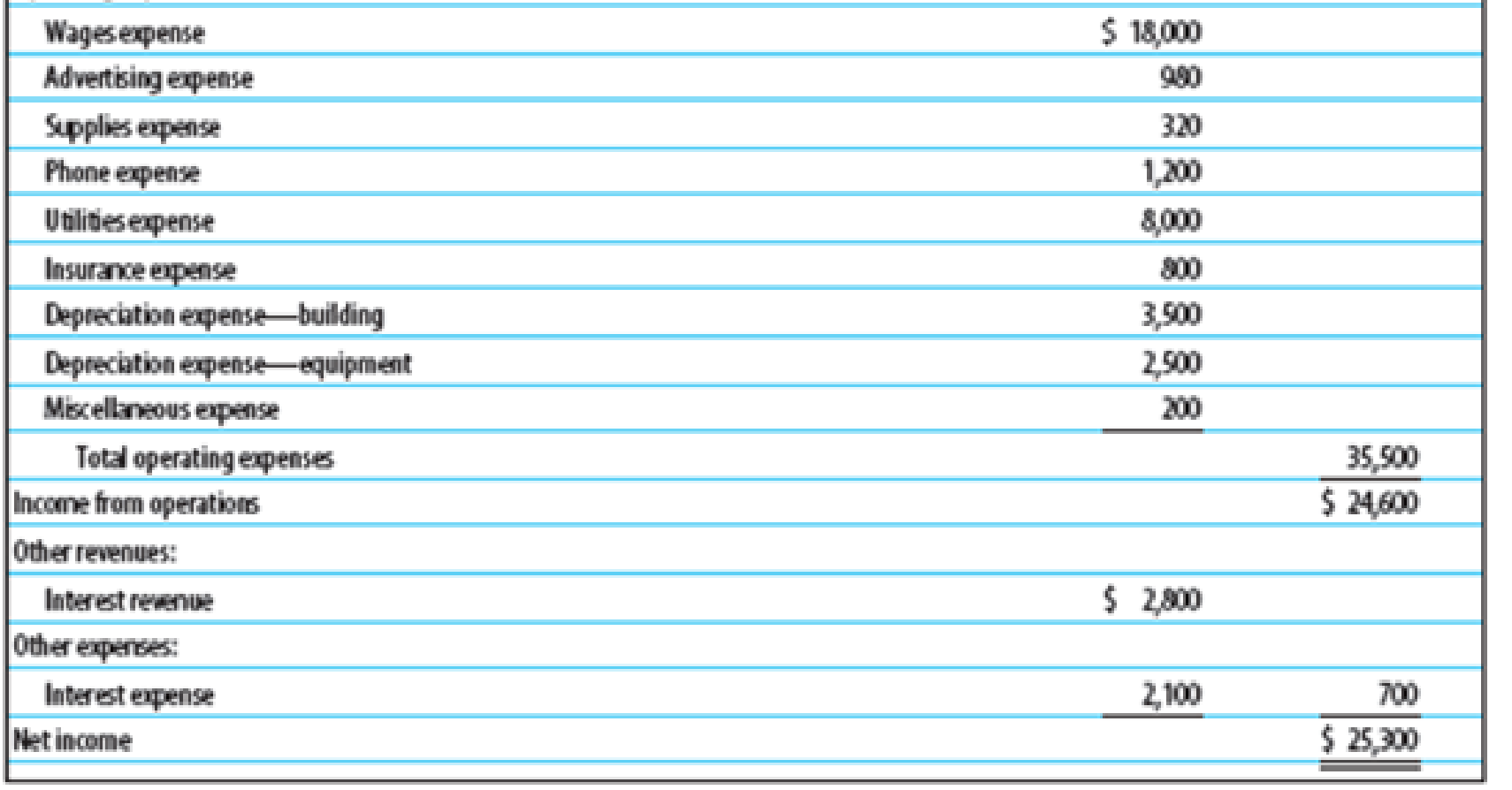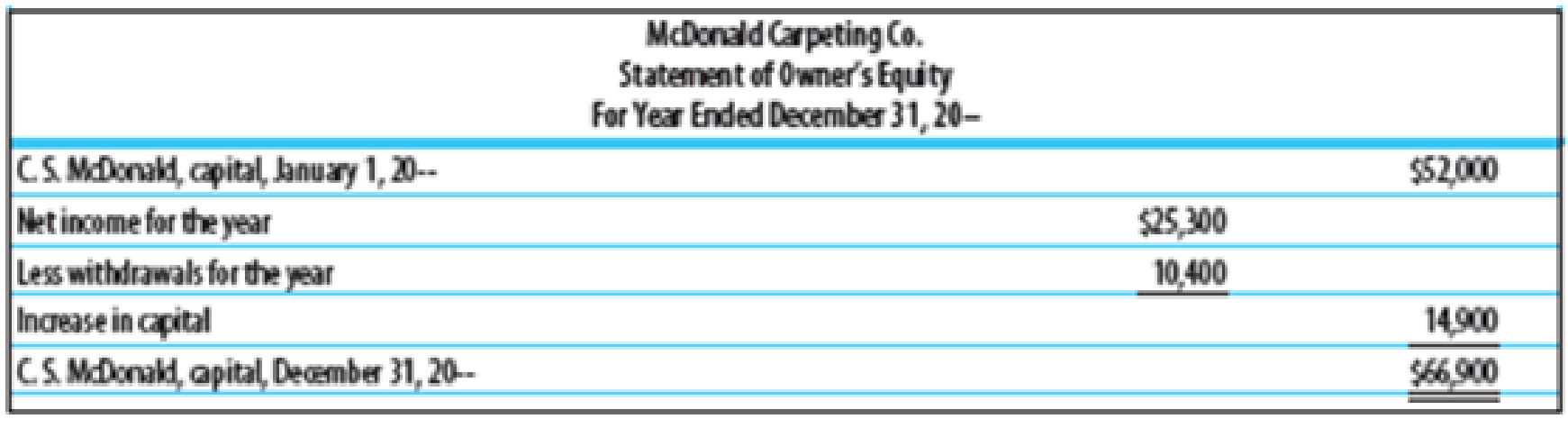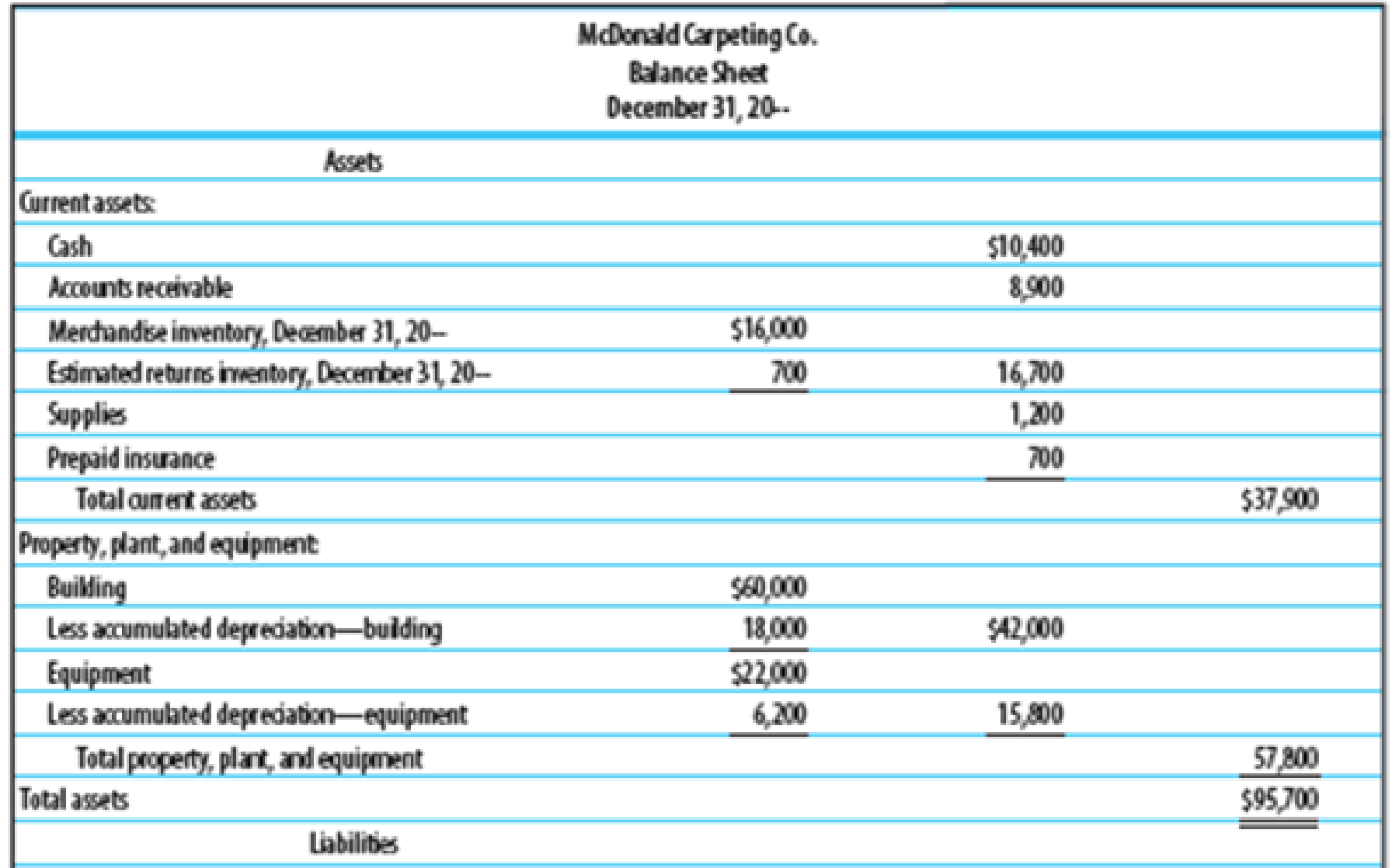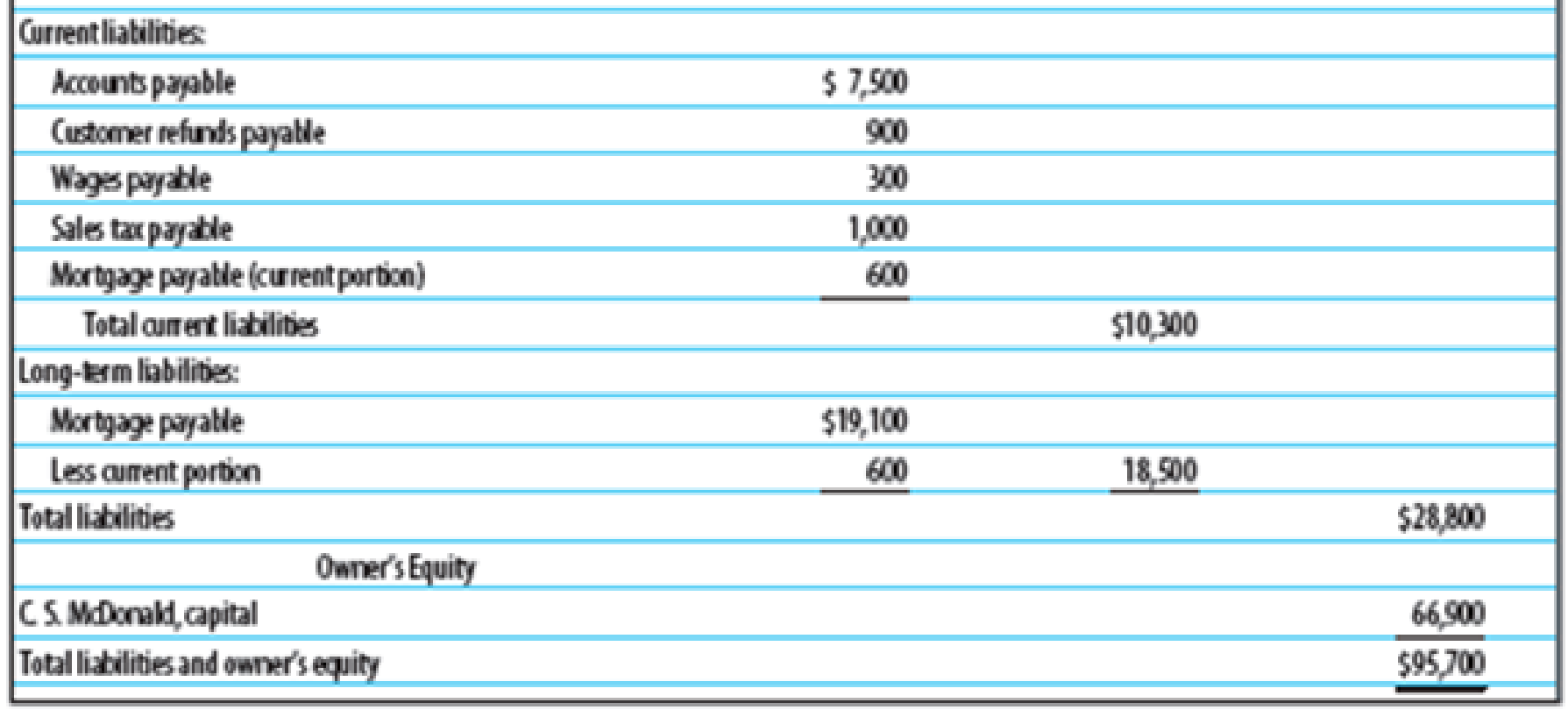To determine Prepare the following financial ratios. 1. 1. Working capital 2. 2. Current ratio 3. 3. Quick ratio 4. 4. Return on owner’s equity 5. 5. Accounts receivable turnover and average number of days require to collect receivables. 6. 6. Inventory turnover and average number of days required to sell inventory Explanation Ratio analysis: The analysis of a company using the financial ratios and comparing its trends and measure its performance within the company and the companies of the industry is known as ratio analysis. The main categories of ratio analyses are liquidity ratios, profitability ratios, activity ratios, and leverage ratios. 1. Compute working capital. Working capital =Current assets Current liabilites=$37,900 $10,300=$27,600

2.

Compute current ratio.

Current ratio =Current assetsCurrent liabilities=$37,900$10,300=3.68to1

3.

Compute quick ratio.

Quick ratio =Quick assetsCurrent liabilites=($10,400+$8,900)$10,300=1.87to 1 4. Compute return on owners’ equity. Return on owners' equity=Net incomeAverage owners' equity=$25,300($52,000+$66,900)2=$25,300$59,450=42.6%

5

Still sussing out bartleby?

Check out a sample textbook solution.

See a sample solution

The Solution to Your Study Problems

Bartleby provides explanations to thousands of textbook problems written by our experts, many with advanced degrees!

Get Started

What are the benefits of retaining customers?

Foundations of Business (MindTap Course List)

Trace the flow of accounting information through the accounting system.

College Accounting, Chapters 1-27 (New in Accounting from Heintz and Parry)

BOND VALUATION An investor has two bonds in her portfolio. Bond C and Bond Z. Each bond matures in 4 years, has...

Fundamentals of Financial Management, Concise Edition (with Thomson ONE - Business School Edition, 1 term (6 months) Printed Access Card) (MindTap Course List)